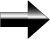How To Master Exponents

What Is An Exponent?

an = a * a * … a.  There are n factors of a here.  “a” is called the “base”, and n is the exponent.  For example, 73 = 7 * 7 * 7.  There are integer, rational, and literal exponents.

The Basic Laws Of Exponents

Anything raised to the zero power is one (but 00 is undefined). That is:

a0 = 1.  100 = 1, 1254350 = 1

Negative Exponents

When you raise a number to negative one it inverts the number. That is:

a-1 = 1/a.  For example, 4-1 = ¼, and 234-1 = 1/234

Fractional Exponents

Raising a number by a fraction actually takes a root of the number.  For example, a1/2 = √ a, and a1/3 = 3√ a.  If we have an expression such as a2/3, this is simply 3√(a2).  74/3 = 3√(74).  a(15/3) = 3√(a15).

The numerator stays as the exponent and the denominator becomes the root.

The Main Laws Of Exponents

Now that we understand the basic rules of exponents, we can begin mastering the rest.

The Product Law: am an = a(m+n)

The Quotient Law: am/an = a(m-n), and a cannot equal zero.  We can think of this in terms of multiplying a negative exponent.  Remember, a negative exponent is the reciprocal of its positive counterpart.  am / an = am * a-n.  When you move an exponent from the numerator to denominator or the denominator to the numerator, the sign of the exponent changes.  So the quotient law IS the product law, using the fact that a-1 = 1/a.  When you divide exponents, you are adding negatives (or subtracting).

The Power Law:  (am)n = am*n.  This is ALSO the product rule.  Think about it this way.  (a2)4 = a2 * a2 * a2 * a2, which, by the product law, is a8 (we add the exponents, since the bases are the same.)  Understanding the Product Law is a key to understanding exponents in general.

The Distributive Laws:   (ab)n = anbn, and (a/b)n = an/ bn, where b cannot equal zero.  Again, this is just using the fact that a-1 = 1/a.  This fact is the bridge between multiplication and division of exponents.

Main Algebra Page